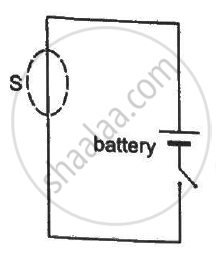Department of Pre-University Education, KarnatakaPUC Karnataka Science Class 12
Share

# A Closed Surface S is Constructed Around a Conducting Wire Connected to a Battery and a Switch in the Following Figure. - Physics

ConceptElectric Field Introduction of Electric Field

#### Question

A closed surface S is constructed around a conducting wire connected to a battery and a switch in the following figure. As the switch is closed, the free electrons in the wire start moving along the wire. In any time interval, the number of electrons entering the closed surface S is equal to the number of electrons leaving it. On closing the switch, the flux of the electric field through the closed surface
(a) is increased
(b) is decreased
(c) remains unchanged
(d) remains zero#### Solution

(c) remains unchanged
(d) remains zero
Initially, there is no charge in the closed surface. As the wire is neutral, the flux initially is zero. Now, if we connect the battery and a current flows through it, the flux remains zero, as the number of electrons entering the surface is equal to number of electrons leaving. That is, net charge enclosed is zero and so is the flux.

Is there an error in this question or solution?

#### APPEARS IN

HC Verma Solution for Concepts of Physics - Vol. 2 (2018 to Current)
Chapter 8: Gauss’s Law
MCQ | Q: 7 | Page no. 140
Solution A Closed Surface S is Constructed Around a Conducting Wire Connected to a Battery and a Switch in the Following Figure. Concept: Electric Field - Introduction of Electric Field.
S